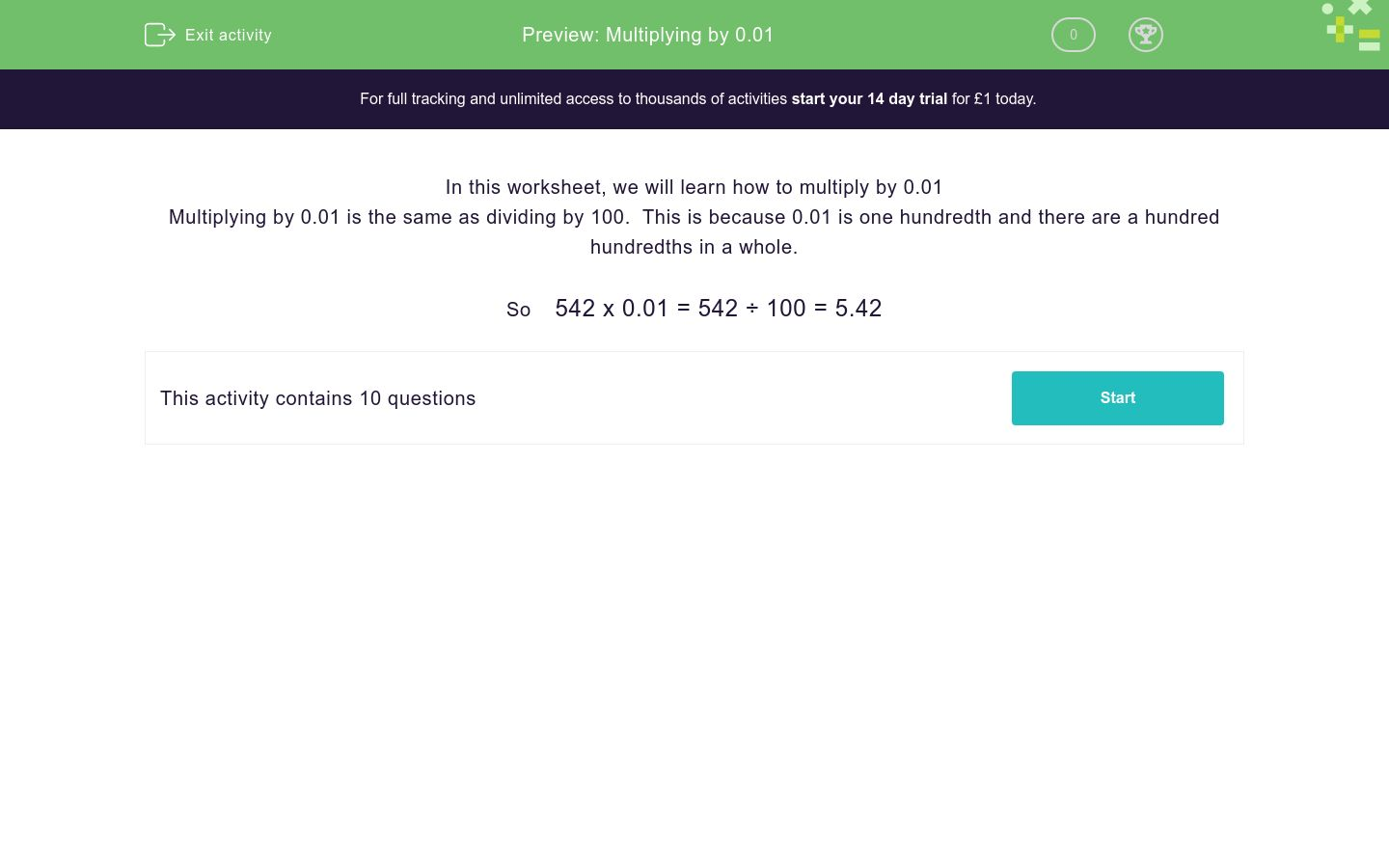# Multiplying by 0.01

In this worksheet, students multiply numbers by 0.01.Key stage:  KS 3

Curriculum topic:   Number

Curriculum subtopic:   Use Four Operations for All Numbers

Difficulty level:### QUESTION 1 of 10

In this worksheet, we will learn how to multiply by 0.01

Multiplying by 0.01 is the same as dividing by 100.  This is because 0.01 is one hundredth and there are a hundred hundredths in a whole.

So    542 x 0.01 = 542 ÷ 100 = 5.42

Find the value of:

7548 × 0.01 = ___________

Find the value of:

2619 × 0.01 = ___________

Work out:

8973 × 0.01 = ___________

Work out:

7460 × 0.01 = ___________

What is...?

7300 × 0.01 = ___________

Find the value of:

663 × 0.01 = ___________

Work out:

53 × 0.01 = ___________

Find the value of:

8666 × 0.01 = ___________

Find the value of:

1694 × 0.01 = ___________

Find the value of:

391 × 0.01 = ___________

• Question 1

Find the value of:

7548 × 0.01 = ___________

75.48
• Question 2

Find the value of:

2619 × 0.01 = ___________

26.19
• Question 3

Work out:

8973 × 0.01 = ___________

89.73
• Question 4

Work out:

7460 × 0.01 = ___________

74.6
• Question 5

What is...?

7300 × 0.01 = ___________

73
• Question 6

Find the value of:

663 × 0.01 = ___________

6.63
• Question 7

Work out:

53 × 0.01 = ___________

0.53
• Question 8

Find the value of:

8666 × 0.01 = ___________

86.66
• Question 9

Find the value of:

1694 × 0.01 = ___________

16.94
• Question 10

Find the value of:

391 × 0.01 = ___________

3.91
---- OR ----

Sign up for a £1 trial so you can track and measure your child's progress on this activity.

### What is EdPlace?

We're your National Curriculum aligned online education content provider helping each child succeed in English, maths and science from year 1 to GCSE. With an EdPlace account you’ll be able to track and measure progress, helping each child achieve their best. We build confidence and attainment by personalising each child’s learning at a level that suits them.

Get started